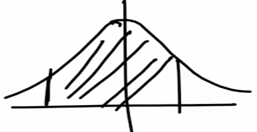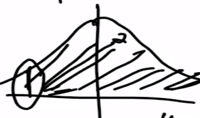Need Help?

Subscribe to Probability

###### \${selected_topic_name}
• Notes

The life in hours of a 75 -watt light bulb is known to be
normally distributed with $\sigma=25$ hours. A random sample of
20 bulbs has a mean life of $\overline{x}=1014$ hours.

(a) Construct a $95\%$ two-sided confidence interval on the
mean life.
(b) Construct a $95\%$ lower-confidence bound on the mean
life.

$\sigma=25, n=20, \overline{x}=1014$

(a) $95\%$ CI Two sided

(b) $95\%$ CI lower-confidence bound(a) $\alpha=1-0.95=0.05$

$\overline{x}-Z_\frac{\alpha}{2}\left(\frac{\sigma}{\sqrt{n}}\right)\leq\mu \leq \overline{x}+Z_\frac{\alpha}{2}\left(\frac{\sigma}{\sqrt{n}}\right)$

$\rightarrow \frac{\alpha}{2}=0.025$

$Z_{0.025}=1.96$

$1014-1.96 \times \frac{25}{\sqrt{20}} \leq \mu \leq 1014+1.96\left(\frac{25}{\sqrt{20}}\right)$

$1003 \leq \mu \leq 1025$$u=\infty$

(b) $\overline{x}-Z_{\alpha} \frac{\sigma}{\sqrt{n}} \leq \mu$

$Z_{\alpha}=Z_{0.05}$

$Z=1.65$

$1014-1.65 \times \frac{25}{\sqrt{20}} \leq \mu$

$1005 \leq \mu$

Suppose that in first Exercise we wanted to be $95\%$
confident that the error in estimating the mean life is less than
five hours. What sample size should be used?

$95 \mathrm{CI} \rightarrow E=5$

$\alpha=0.05$

$\sigma=25$

$n ?!$

$E=5$

$n=\left(\frac{Z_{\frac{\alpha}{2}} \sigma}{E}\right)^{2}$

$\frac{\alpha}{2}=0.025 \rightarrow Z_{0.025}=1.96$

$n=\left(\frac{1.96 \times 25}{5}\right)^{2}$

$=96.04$

$n=97$